# The FASTCLUS Procedure

### Computational Resources

Let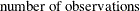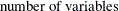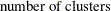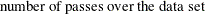#### Memory

The memory required is approximately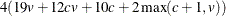bytes.

If you request the DISTANCE option, an additional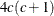bytes of space is needed.

#### Time

The overall time required by PROC FASTCLUS is roughly proportional to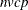if c is small with respect to n.

Initial seed selection requires one pass over the data set. If the observations are in random order, the time required is roughly proportional to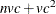unless you specify REPLACE=NONE. In that case, a complete pass might not be necessary, and the time is roughly proportional to, where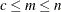.

The DRIFT option, each iteration, and the final assignment of cluster seeds each require one pass, with time for each pass roughly proportional to.

For greatest efficiency, you should list the variables in the VAR statement in order of decreasing variance.